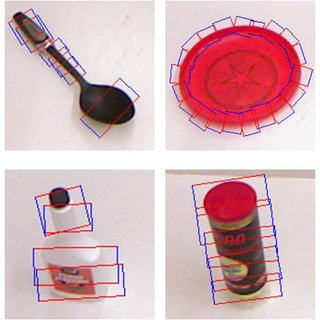$$\newcommand{\dint}{\mathrm{d}} \newcommand{\vphi}{\boldsymbol{\phi}} \newcommand{\vpi}{\boldsymbol{\pi}} \newcommand{\vpsi}{\boldsymbol{\psi}} \newcommand{\vomg}{\boldsymbol{\omega}} \newcommand{\vsigma}{\boldsymbol{\sigma}} \newcommand{\vzeta}{\boldsymbol{\zeta}} \renewcommand{\vx}{\mathbf{x}} \renewcommand{\vy}{\mathbf{y}} \renewcommand{\vz}{\mathbf{z}} \renewcommand{\vh}{\mathbf{h}} \renewcommand{\b}{\mathbf} \renewcommand{\vec}{\mathrm{vec}} \newcommand{\vecemph}{\mathrm{vec}} \newcommand{\mvn}{\mathcal{MN}} \newcommand{\G}{\mathcal{G}} \newcommand{\M}{\mathcal{M}} \newcommand{\N}{\mathcal{N}} \newcommand{\S}{\mathcal{S}} \newcommand{\diag}{\mathrm{diag}(#1)} \newcommand{\diagemph}{\mathrm{diag}(#1)} \newcommand{\tr}{\text{tr}(#1)} \renewcommand{\C}{\mathbb{C}} \renewcommand{\R}{\mathbb{R}} \renewcommand{\E}{\mathbb{E}} \newcommand{\D}{\mathcal{D}} \newcommand{\inner}{\langle #1 \rangle} \newcommand{\innerbig}{\left \langle #1 \right \rangle} \newcommand{\abs}{\lvert #1 \rvert} \newcommand{\norm}{\lVert #1 \rVert} \newcommand{\two}{\mathrm{II}} \newcommand{\GL}{\mathrm{GL}} \newcommand{\Id}{\mathrm{Id}} \newcommand{\grad}{\mathrm{grad} \, #1} \newcommand{\gradat}{\mathrm{grad} \, #1 \, \vert_{#2}} \newcommand{\Hess}{\mathrm{Hess} \, #1} \newcommand{\T}{\text{T}} \newcommand{\dim}{\mathrm{dim} \, #1} \newcommand{\partder}{\frac{\partial #1}{\partial #2}} \newcommand{\rank}{\mathrm{rank} \, #1}$$

# Grasping Regression

## Introduction

Knowing how to grab an object is an essential skill for robots, it allows manipulation, kit creation or even automated sorting ... To be efficient in an open world, a robot must be able to catch any kind of object, even unfamiliar objects. Use of deep learning then seems interesting because of its high quality of generalization. In this mini-project, we therefore propose to create a model to determine the parameters of grasping on an object. For this, we will use the Cornell databaseCornell database including grasping areas for each object
which includes a collection of images (750 images) as well as the associated grasping parameters.

In order to be able to carry out your python project, you will use a jupyter file.

## HomeWork

The work to be done is detailed by following the jupyter file: Automatic Grasping. Below, the different points you will discuss:

1. Creation of a neural network for grasping rectangle prediction
2. Network performance assessment
3. Writing a Custom Loss Function
4. Writing of a data augmentation class to improve generalization capabilities

## Deliverables

The Deliverables to be provided are:

• A pdf report of about 10 pages explaining the subject, the choice of your approach, the implementation, the tests carried out, and the careful analysis of the results.
• Commented Python files.
The report and the work (implemented in Python) will lead to the evaluation score. It is important to note that the originality of the approach and the relevance of the analysis of the results will have a great importance in the assessment.
To help you, here is a notebook detailing the different stages of a deep learning project (RESSOURCE). Do not hesitate to use this as inspiration when creating networks in the future.